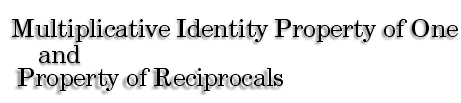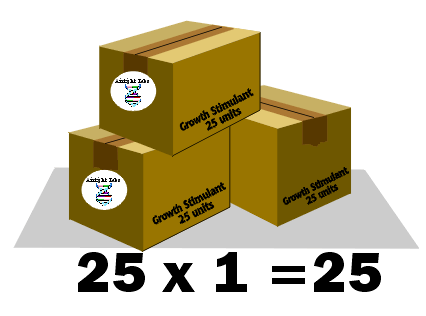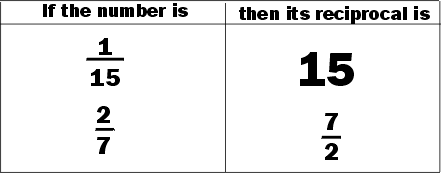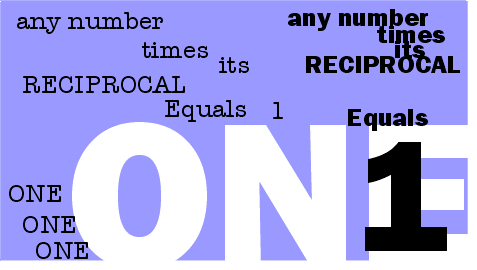Professor Monique Penseur has created a growth stimulating bacteria for grape vines. She decides to package her growth stimulating bacteria in 25- unit boxes for distribution to the vineyards which have agreed to test the bacteria on their grapevines.If she sends each vineyard 1 box of the bacteria, how many units of bacteria does each vineyard receive?The answer can be found by multiplying the units per box (25) by the number of boxes (1). 25 × 1 = 25. Any number multiplied by 1 remains the same. One is called the Multiplicative Identity. Multiplying any number by one is an example of the Multiplicative Identity Property of One.

 The Boisson Vineyard in Burgundy is one of the vineyards which has decided to test the effect of the bacteria on the growth of their grapevines.A technician at the vineyard adds 1/25 of the box to a vine. How many units of the bacteria is added to the vine? To answer this question, we can multiply the contents of the box (25 units) by the amount used (1/25 of the box). 25 × 1/25 = 1. 25 and 1/25 are known as multiplicative inverses or reciprocals, because when multiplied, the result is one. The reciprocal of any number is just one divided by that number. The Property of Reciprocals states that any non-zero number multiplied by its reciprocal yields a product of one.Suppose Professor Penseur packages
the bacteria in boxes of
10 units each.
The Vinblanc Vineyard orders
1 box and
uses
1/10 of the box.

 How many units of bacteria does the Vinblanc Vineyard use?

Additive Property of Zero     Number Theory Main